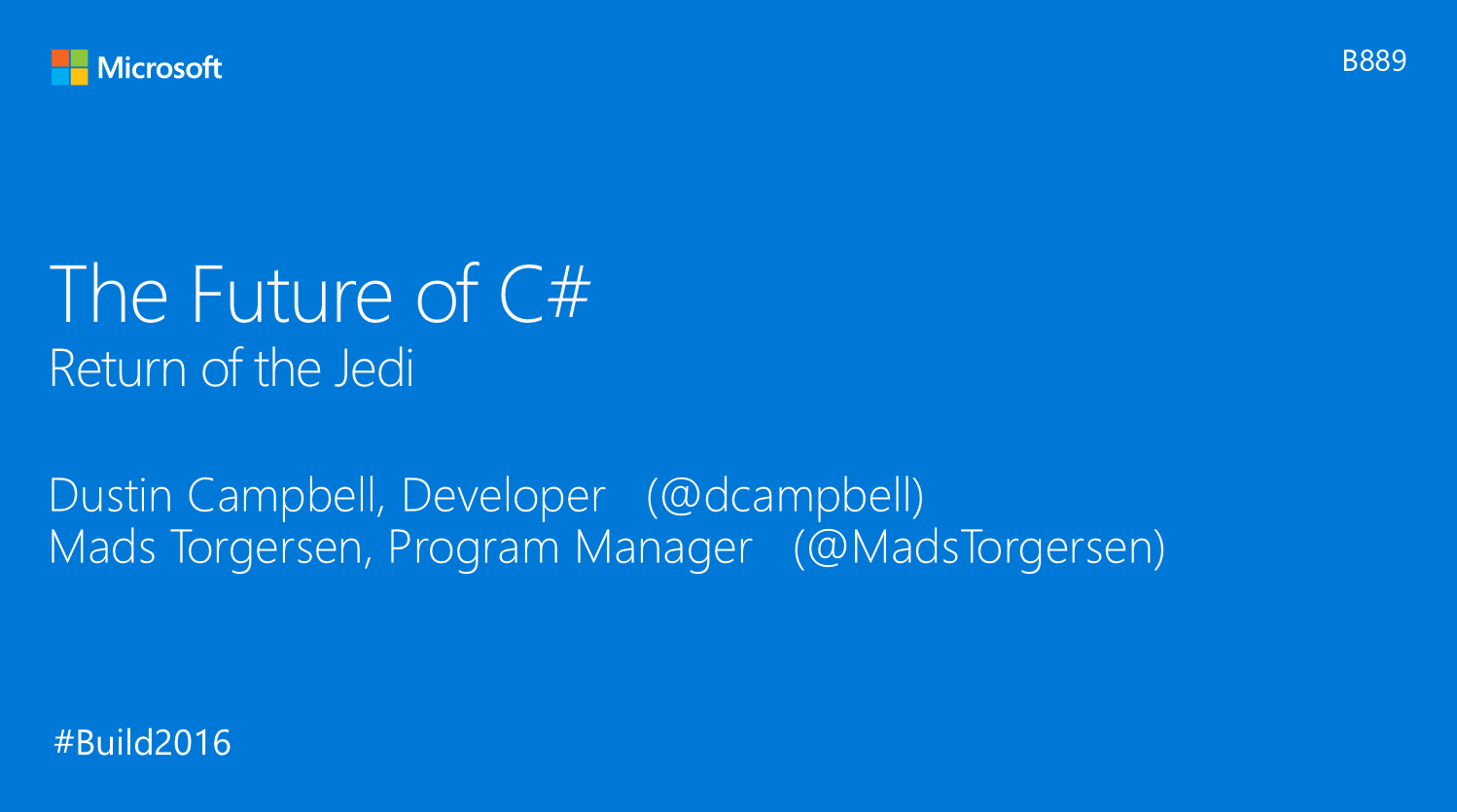### C# 7新語法預覽

• 12280
• 0
• 2016-06-10

Build 2016過後微軟投出了很多讓開發人員感到驚豔的震撼彈，像是Win10未來可以run原生的使用linux指令，Xamrian免費等等。趁著連假也惡補了幾段內容，其中「The Future of C#」談到了一些未來C# 7的新語法，讓人感到滿興奮的，因此在這邊做個簡單的紀錄。※本篇所撰寫的C#7語法皆沒有實際貼到Visual Studio裡面編譯過，只是提出用法說明而已。

## Binary literals

C# 7可以使用2進位的方式來表示數值內容，如同使用0xXX來表示16進位，現在也可以使用0bXX的方式來表示2進位數字。

``````int num1 = 8;
int num2 = 0b1000;
Console.WriteLine(\$"Num1 = {num1}");
Console.WriteLine(\$"num2 = {num2}");
// 結果為
// Num1 = 8
// Num2 = 8``````

## Digit separators

``````// 傳統宣告方式
int num1 = 1000000;
// 當千份號使用
int num2 = 1_000_000;
// 隨便使用
int num3 = 1_00_00_00;
int num4 = 1__000___000;
``````

## Tuples

``````        void GetHeightAndWeight(out int height, out int weight)
{
height = 172;
weight = 80;
}

void OutputBody()
{
int height, weight;
getHeightAndWeight(out height, out weight);
Console.WriteLine(\$"Height = {height}");
Console.WriteLine(\$"Weight = {weight}");
// Height = 172
// Weight = 80
}``````

``````        Tuple<int, int> GetHeightAndWeight()
{
var returnVal = new Tuple<int, int>(172, 80);
return returnVal;
}

void OutputBody()
{
var body = GetHeightAndWeight();
Console.WriteLine(\$"Height = {body.Item1}");
Console.WriteLine(\$"Weight = {body.Item2}");
// Height = 172
// Weight = 80
}``````

``````// 用括弧的方式直接代表Tuples
(int, int) GetHeightAndWeight(){
var returnVal = (172, 80)
return returnVal;
}

void OutputBody(){
var body = GetHeightAndWeight();
WriteLine("Height = {body.Item1}");
WriteLine("Weight = {body.Item2}");
// Height = 172
// Weight = 80
}``````

``````void OutputBody(){
// 用自訂名稱來取代Tuple的Item1, Item2
var body = (Height: 172, Weight: 80);
WriteLine("Height = {body.Height}");
WriteLine("Weight = {body.Weight}");
// Height = 172
// Weight = 80
}``````

## Patten matching

Pattern matching主要是用來減少型別檢查時所需撰寫的程式碼，例如我們有一個object陣列，想要加總陣列裡面的整數部分時，過去我們程式碼會寫成這樣：

``````        static void Main(string[] args)
{
object[] numbers = { "a", 1, 2, "b", new object[] { 3, 4, 5 }};
Console.WriteLine(sum(numbers));
}

static int sum(IEnumerable<object> list)
{
var result = 0;
foreach(var val in list)
{
if (val is int)
{
var num = (int)val;
result += num;
}
}
return result;
}``````

``````if (val is int num)
{
result += num;
}``````

``````        static int sum(IEnumerable<object> list)
{
var result = 0;
foreach(var val in list)
{
switch(val)
{
case int num:
result += num
break;
case IEnumerable<object> l when l.Any(): // 使用when做額外的條件限定
result += sum(l);
break;
}
}
return result;
}``````

## Local functions

``````void func1()
{
// local function
int func2()
{
return 0;
}
var result = func2();
Console.WriteLine(\$"Call func2() = {result}");
// Call func2() = 0
}

void func3()
{
var result = func2(); // 編譯錯誤
}``````

## Ref returns and locals

``````void FuncRef(ref int data)
{
// do something
}``````

``````        static void Main(string[] args)
{
int[] numbers = { 1, 2, 4 };
// 回傳的是參考，不是值
var firstRef = FirtRef(numbers);
}

static ref int FirstRef(ref int[] data)
{
// 回傳第一筆資料的參考
return ref data;
}``````